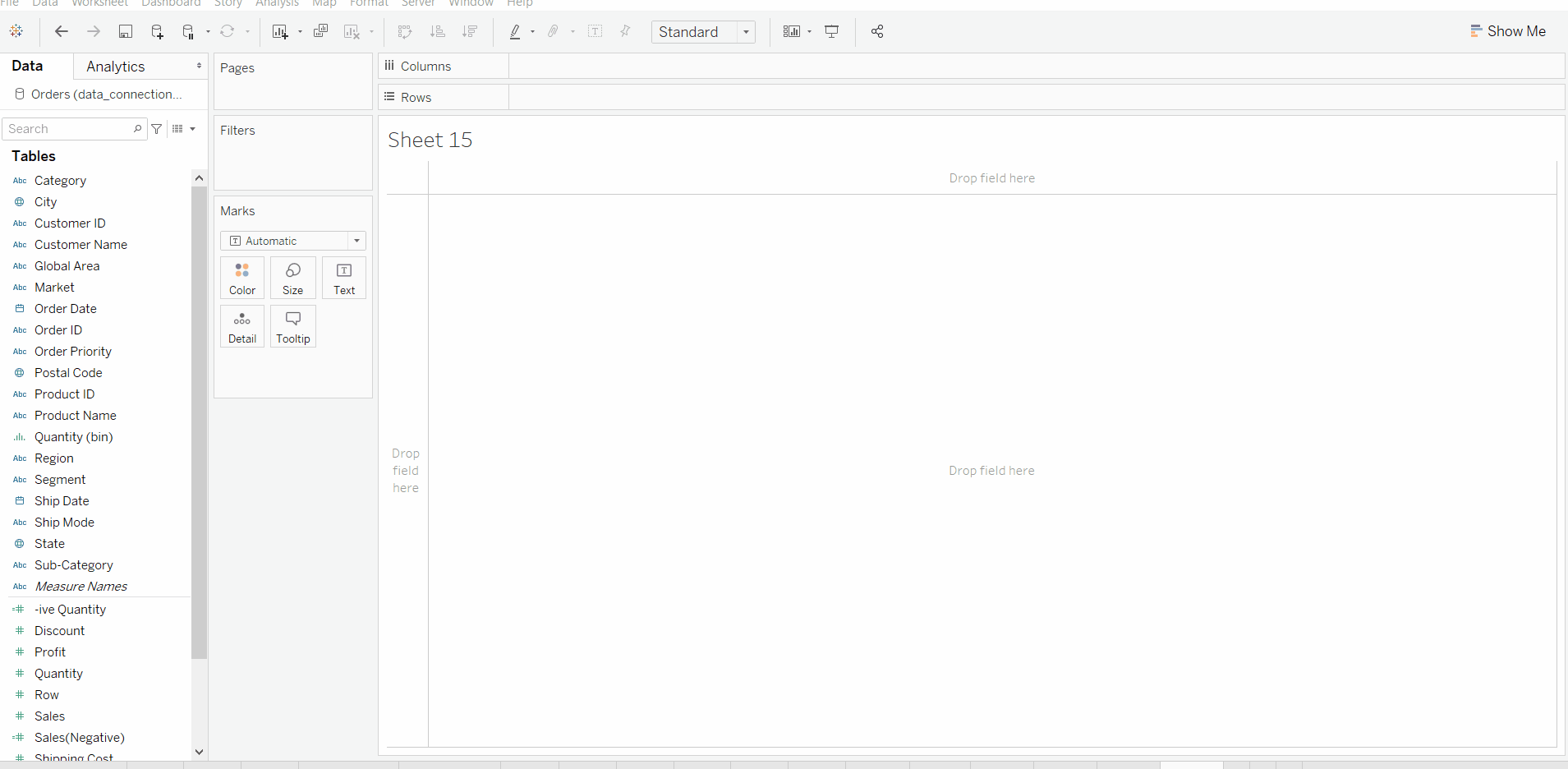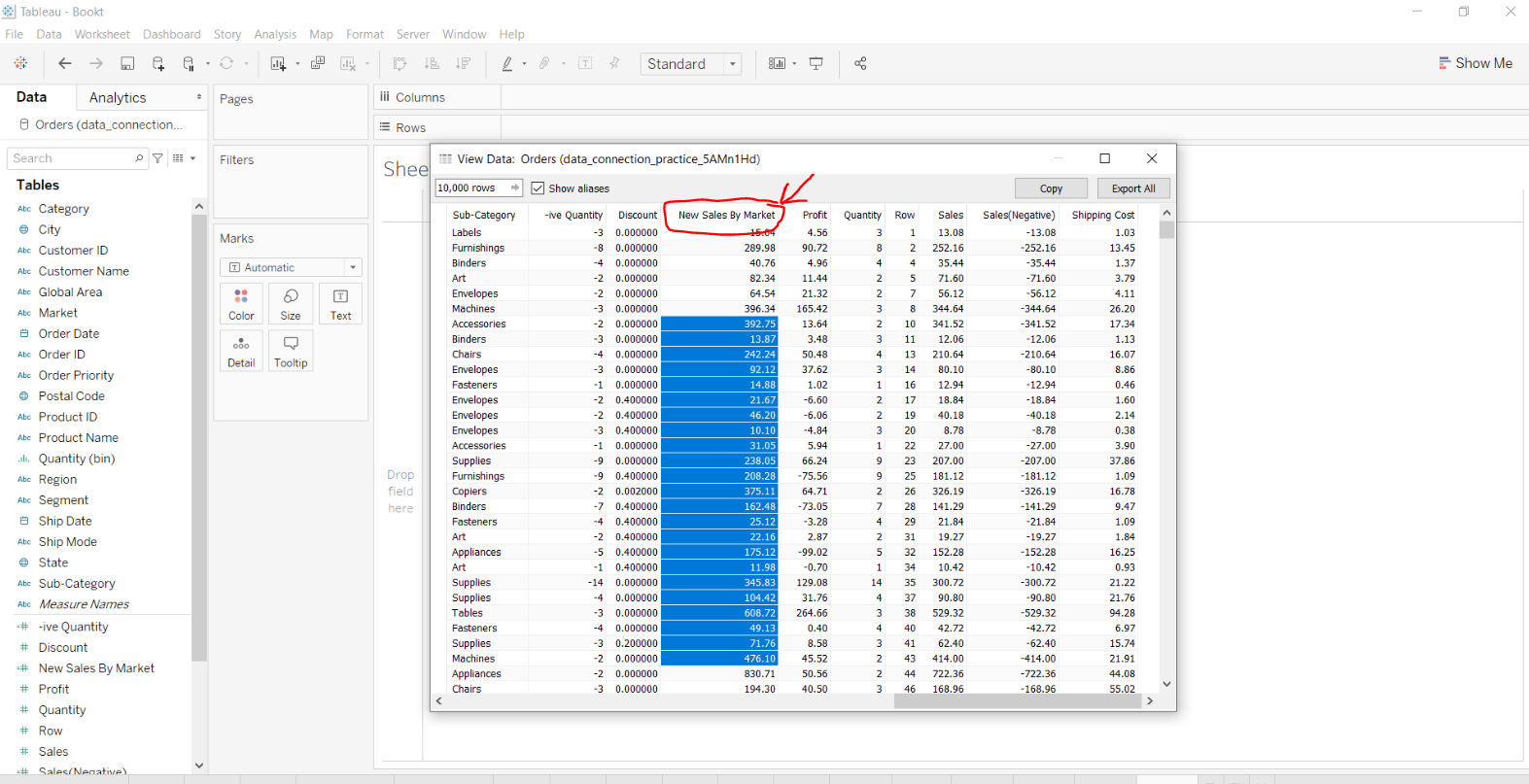Open in App
Not now

# Case Function in Tableau

• Last Updated : 22 Oct, 2020

• Tableau: Tableau is a very powerful data visualization tool that can be used by data analysts, scientists, statisticians, etc. to visualize the data and get a clear opinion based on the data analysis. Tableau is very famous as it can take in data and produce the required data visualization output in a very short time.
• Case Function: Case Function is the part of Logical functions in Tableau. These functions are used to perform the logical test and return the required value when the test expression is true.

#### How CASE function works:

• It starts with CASE function evaluating the 1st logical expression corresponding to set/sequence of values and when the logical expression becomes True, it returns the respective specified value as result.
• If no match is found in logical expression then the value of default return expression is used.
• In case no default value is being mentioned by the user then NULL is returned.

#### Syntax of CASE Function:

This function finds the first that matches the given <expression> and returns the corresponding value as a result.

```CASE [<expression>]
WHEN <expression> THEN <expression>
WHEN <expression> THEN <expression>
ELSE <expression>
END
```

#### Example:

• Dataset used in the given examples is Dataset.
• In this example, we simply create a new calculated field by using the CASE function on some fields.• View new calculated field.• Use in Visualization.#### Advantages of using CASE Function:

• CASE-WHEN statements are easier to write down and comprehend.
• Due to its simplicity, for a user, it’s helpful to avoid making mistakes like referencing the incorrect field.
• CASE-WHEN statements perform faster than IF-ELSE statements.

#### Disadvantages of using CASE Function:

• Usage of CASE-WHEN in Tableau is extremely limited as they can’t perform Boolean logic conditions.
• CASE-WHEN in tableau only compares the expression to the precise values.
• Conditional operators like OR, AND can’t be used with CASE-WHEN.
• Using CASE-WHEN, multiple expressions can’t be evaluated during a single line.
My Personal Notes arrow_drop_up